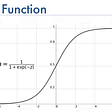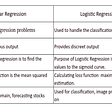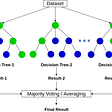# Logistic Regression

With binary logistic regression, the goal is to find a way to separate your two classes. There are a number of ways of visualizing this.

No matter which of these you choose to think of, we can agree logistic regression defines a decision rule

h(x|theta) = sigmoid(x dot theta + b)

and seeks a theta which minimizes some objective function, usually

loss(theta)= ∑ y*log(h(x|theta)) + (1−y)log(1−h(x|theta))

which is obfuscated by a couple clever tricks. It is derived from the intuitive objective function:

loss(theta)= ∑ (y - h(x|theta))

i.e. the number of misclassified x, which makes sense to try to minimize.

# Regularization

In many cases, you wish to regularize your parameter vector theta. This means you want to both minimize the number of misclassified examples while also minimizing the magnitude of the parameter vector. These objectives are in opposition, and so the data scientist needs to decide on the appropriate balance between those objectives using their intuition, or via many empirical tests (e.g. by cross validation).

Let’s rename our previous loss function

loss(theta)= ∑ y*log(h(x|theta)) + (1−y)log(1−h(x|theta))

the basic_loss(theta). Our new, regularized loss function will look like:

loss(theta) = basic_loss(theta) + k * magnitude(theta)

Recall we’re trying to minimize loss(theta) which means we’re applying downwards pressure on both the number of mistakes we make as well as the magnitude of theta. In the above loss function, k is a hyperparameter which modulates the tradeoff of how much downwards pressure we apply to the error of the classifier defined by theta versus the magnitude of theta. Therefore, k encodes our prior beliefs, our intuitions, as to how the process we’re modeling is most likely to behave.

# Norms

Now on to the interesting part. It turns out there is not one, but many ways of defining the magnitude (also called the Norm) of a vector. The most commonly used norms are the p-norms, which have the following character:

For p = 1 we get the L1 norm (also called the taxicab norm), for p = 2 we get the L2 norm (also called the Euclidean norm), and as p approaches ∞ the p-norm approaches the infinity norm (also called the maximum norm). The Lp nomenclature comes from the work of a mathematician called Lebesgue.

Returning to our loss function, if we choose L1 as our norm,

loss(theta) = basic_loss(theta) + k * L1(theta)

is called “the LASSO”. If we choose the L2 norm,

loss(theta) = basic_loss(theta) + k * L2(theta)

is called Ridge Regression (which also turns out to have other names). If we decide we’d like a little of both,

loss(theta) = basic_loss(theta) + k(j*L1(theta) + (1-j)L2(theta))

is called “Elastic Net”. Notice the addition of a second hyperparameter here. Notice also that ElasticNet encompasses both the LASSO and Ridge, by setting hyperparameter j to 1 or 0.

# On the Naming of Algorithms

Academia has a complicated incentive structure. One aspect of that incentive structure is that it is desirable to have a unique name for your algorithmic invention, even when that invention is a minor derivative of another idea, or even the same idea applied in a different context. Take, for example, Principal Component Analysis.

PCA was invented in 1901 by Karl Pearson, as an analogue of the principal axis theorem in mechanics; it was later independently developed and named by Harold Hotelling in the 1930s. Depending on the field of application, it is also named the discrete Kosambi-Karhunen–Loève transform (KLT) in signal processing, the Hotelling transform in multivariate quality control, proper orthogonal decomposition (POD) in mechanical engineering, singular value decomposition (SVD) of X (Golub and Van Loan, 1983), eigenvalue decomposition (EVD) of XTX in linear algebra, factor analysis, Eckart–Young theorem (Harman, 1960), or Schmidt–Mirsky theorem in psychometrics, empirical orthogonal functions (EOF) in meteorological science, empirical eigenfunction decomposition (Sirovich, 1987), empirical component analysis (Lorenz, 1956), quasiharmonic modes (Brooks et al., 1988), spectral decomposition in noise and vibration, and empirical modal analysis in structural dynamics.

That’s 14 unique names for PCA.

I’m writing this article because the question at the top of this piece was quite hard to find an answer to online. I ended up finding part of the answer in The Elements of Statistical Learning (written by the authors of the regularization methods above, in fact) and the rest from Karen Sachs.

I personally believe that the words Lasso, Ridge, and ElasticNet should not exist. We should call these for what they are: L1-regularization, L2-regularization, and mixed-L1-L2-regularization. A mouthful, sure, but dramatically more unambiguous.

The organization of scikit-learn may have been what caused my confusion in the first place. When looking through their list of regression models, LASSO is its own class, despite the fact that the logistic regression class also has an L1-regularization option (the same is true for Ridge/L2). This is unexpected from a python library, since one of the core dogmas of python is:

`There should be one - and preferably only one - obvious way to do it`

# Comparing regularization techniques — Intuition

Now that we have disambiguated what these regularization techniques are, let’s finally address the question: What is the difference between Ridge Regression, the LASSO, and ElasticNet?

The intuition is as follows:

Consider the plots of the abs and square functions.

When minimizing a loss function with a regularization term, each of the entries in the parameter vector theta are “pulled” down towards zero. Think of each entry in theta lying on one the above curves and being subjected to “gravity” proportional to the regularization hyperparameter k. In the context of L1-regularization, the entries of theta are pulled towards zero proportionally to their absolute values — they lie on the red curve.
In the context of L2-regularization, the entries are pulled towards zero proportionally to their squares — the blue curve.

At first, L2 seems more severe, but the caveat is that, approaching zero, a different picture emerges:

The result is that L2 regularization drives many of your parameters down, but will not necessarily eradicate them, since the penalty all but disappears near zero. Contrarily, L1 regularization forces non-essential entries of theta all the way to zero.

Adding ElasticNet (with 0.5 of each L1 and L2) to the picture, we can see it functions as a compromise between the two. One can imagine bending the yellow curve towards either red or blue by tuning the hyperparameter j.

# Comparing regularization techniques — In Practice

There are a number of reasons to regularize regressions. Typically, the goal is to prevent overfitting, and in that case, L2 has some nice theoretical guarantees built into it. Another purpose for regularization is often interpretability, and in that case, L1-regularization can be quite powerful.

In my work, I deal with a lot of proteomics data. In proteomics data, you have counts for some number of proteins for some number of patients — a matrix of patients by protein abundances, and the goal is to understand which proteins play a role in separating your patients by label.

This is an Ovarian Cancer dataset. Let’s first perform logistic regression with an L2-penalty and try to understand how the cancer subtypes are distinct. This is a plot of the learned theta:

You see that many, if not all proteins are registering as significant.
Now consider the same approach but with L1-regularization:

A much clearer picture emerges of the relevant proteins to each Ovarian Cancer subtype. This is the power of L1-regularization for interpretability.

# Conclusion

Regularization can be very powerful, but it’s somewhat under-appreciated, partially, I think, because the intuitions aren’t always well explained.
The ideas are mostly very simple, but not terribly well documented much of the time. I hope this article helps mend that deficit.

Thanks to Karen Sachs for explaining the intuitions behind these norms many years ago.

Thanks also to the developers of scikit-learn. Despite the occasional unintuitive APIs, the code you make available is invaluable to data scientists like myself.

--

--

--

## More from Alex Lenail

conscious mammalian organism, fanatical tea snob.

Love podcasts or audiobooks? Learn on the go with our new app.

## How Bias and Variance help to classify Underfitting and Overfitting of Model## Hopper’s Data Science DNA: The Tenets That Define Our Team## 6 Things To Keep In Mind While Writing SOP For A Data Science MS Programme## Guide To The PDHPE Long Response## Discover CRISP-DM Framework in Data Analytics## Speech Recognition using IBM’s Speech-to-Text API## Automate SAP Report Extraction with Pyautogui## 3 Reasons Why Data Scientists Leave Their Jobs and How to Build A Fulfilling Career in 2022## Alex Lenail

conscious mammalian organism, fanatical tea snob.

## Logistic Regression## Choosing the Right Algorithm: Logistic Regression vs Linear Regression## Why Random Forest performs better than normal Decision Tree & Logistic Regression when there is an…